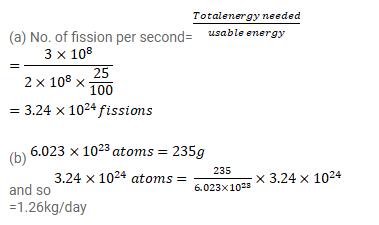# A town has a population of 1 million.

Question:

A town has a population of 1 million. The average electric power needed per person is 300 W.A reactor is to be designed to supply power to this town. The efficiency with which thermal power is converted into electric power is aimed at $25 \%$.

(a) Assuming $200 \mathrm{MeV}$ of thermal energy to come from each fission event on an average, find the number of events that should take place every day.

(b) Assuming the fission to take place largely through ${ }^{235} \mathrm{U}$, at what

Solution: